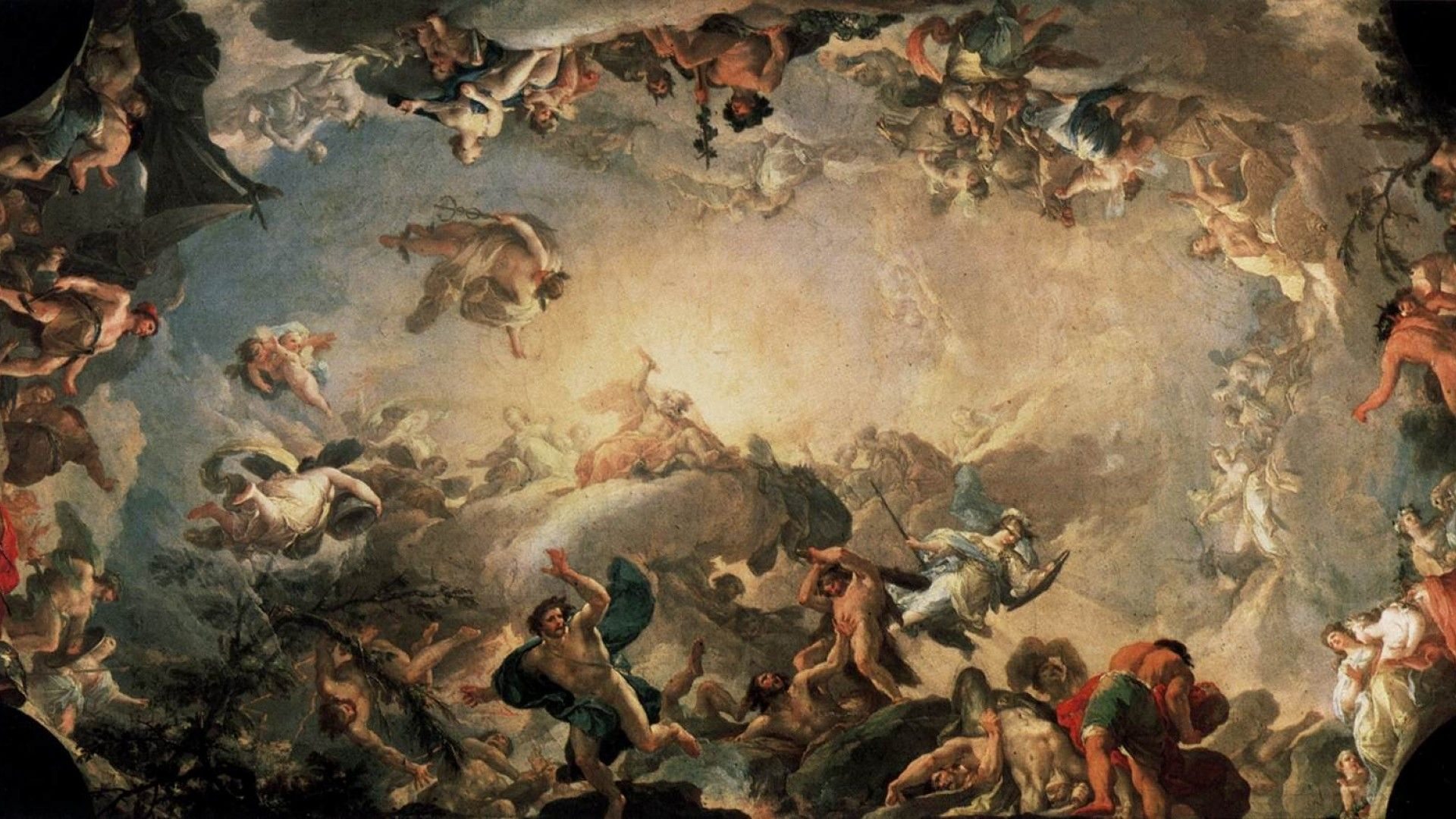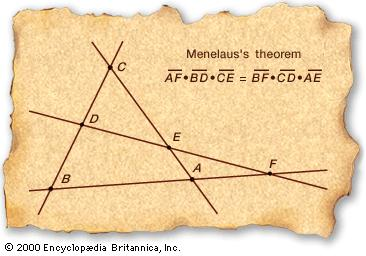TheDomeENTER THE WORLD OF MYTHS AND LEGENDS## Menelaus of AlexandriaMenelaus of Alexandria (Greek: Μενέλαος, Menelaos; c. 70 – 140 CE) was a Greek mathematician and astronomer, the first to recognize geodesics on a curved surface as natural analogs of straight lines.

Menelaus of Alexandria, (flourished 1st century ad, Alexandria and Rome) Greek mathematician and astronomer who first conceived and defined a spherical triangle (a triangle formed by three arcs of great circles on the surface of a sphere).Menelaus’s most important work is Sphaerica, on the geometry of the sphere, extant only in an Arabic translation. In Book I he established the basis for a mathematical treatment of spherical triangles analogous to Euclid’s treatment of plane triangles. Furthermore, he originated the use of arcs of great circles instead of arcs of parallel circles on the sphere, a major turning point in the development of spherical trigonometry. Book II established theorems whose principal interest is their (unstated) application to problems in spherical astronomy. Book III, the last, concentrates on spherical trigonometry and introduces Menelaus’s theorem. The form of this theorem for plane triangles, well known to his contemporaries, was expressed as follows: if the three sides of a triangle are crossed by a straight line (one of the sides is extended beyond its vertices), then the product of three of the nonadjacent line segments thus formed is equal to the product of three other line segments (see the figure below).Although Book III contains the first-known extension of Menelaus’s theorem for spherical triangles, it is quite possible that the theorem was already known and Menelaus simply transmitted it to later generations. In the form stated in Book III, the theorem became of fundamental importance in spherical trigonometry and astronomy, and the theorem has since been known by his name. Other works are attributed to him, including one on setting times of the signs of the zodiac, one (in six books) on chords in a circle, and one (in three books) on elements of geometry, but his only extant work is Sphaerica. Menelaus was not just a theoretical astronomer, as attested by the Almagest where Ptolemy (c. ad 100–170) reports Menelaus’s observations of lunar occultations of stars.



### Our Mobile Application

Check out Our Mobile Application "Ancient Greece Reloaded"